Please start any new threads on our new site at https://forums.sqlteam.com. We've got lots of great SQL Server experts to answer whatever question you can come up with.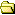All Forums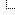SQL Server 2005 Forums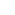Transact-SQL (2005)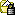6 decimal place limit

Author  Topic

friction
Starting Member

5 Posts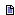Posted - 2007-11-13 : 23:20:46 Hi Guys, here's one that's doing my head in:SITUATIONI have a table with field names such as ,  and , each with the datatype decimal(38,19).PROBLEMI have a view in the server management studio that contains:  / ( - ) AS Result. However the result is only 6 decimal places long, when it should be 19 decimal places long.THE PLOT THICKENS and EXAMPLE CODE`SELECT CompanyCode, PeriodEndDate, , , ,  / ( - ) AS Result, 6716000.000 / (183239000.000 - 141542000.000) AS Result2, 6716000.000000000000000000 / (183239000.000000000000000000 - 141542000.000000000000000000) AS Result3FROM dbo.RawDataWHERE (CompanyCode = 'AAC') AND (PeriodEndDate = CONVERT(DATETIME, '2005-12-30 00:00:00', 102))`with a result of:`AAC30/12/2005 12:00:00 AM6716000.0000000000000000000183239000.0000000000000000000141542000.00000000000000000000.1610660.161066743410796930.1610667434107`Notice that the exact same calculation using .000 at the end of each integer gives me a result with MORE precision than using 18 zero's after the decimal point?I'VE ALSO TRIEDI've also tried using CAST() around each reference to a field, and around the end result, but all it does is pad the 6 decimal places out with zero's on the end of it.WHY AM I USING THESE DATATYPES?I'm forced to use the DECIMAL(38,19) datatype because some of the values are 19 digits long with no decimal, and others are zero with 19 digits of fractional component, and I can't have the loss of accuracy that comes with using floats.Does anyone know what's going on?

PeterNeo
Constraint Violating Yak Guru

357 PostsPosted - 2007-11-13 : 23:51:27 Try this CONVERT(DECIMAL(38, 19),  / ( - )) AS Result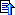dataguru1971
Master Smack Fu Yak Hacker

1464 PostsPosted - 2007-11-14 : 00:01:16 quote:Originally posted by PeterNeoTry this CONVERT(DECIMAL(38, 19),  / ( - )) AS ResultThat won't do it...should have tested :) Check result of R2 below..`Create Table #Dec (num1 numeric(38,19) not null, num2 numeric(38,19) not null, num3 numeric(38,19) not null)Insert Into #Dec (num1,num2,num3)Select 6716000,183239000,141542000Select num1 / (num2-num3) as NOCHANGE,convert(float,num1) / (convert(float,num2) - convert(float,num3)) as R1,convert(numeric(38,19), num1/(num2-num3)) as R2,(num1 + .0000000000000000000)/((num2 + .00000000000000)-(num3 + .0000000000000000)) as R3FROM #DECDROP Table #DEC`ResultsNochange .161066 R1 0.16106674341079694R2 .1610660000000000000 R3 .161066Not sure what the answer is, but float seems to work best. I tried a number of combinations which only resulted in protracted results at the sixth place.Poor planning on your part does not constitute an emergency on my part.friction
Starting Member

5 PostsPosted - 2007-11-14 : 05:44:33 I'd love to use float, but I can't have values changing even if it's seemingly small like after the 6th decimal place. I just don't understand why it truncates (it doesn't round, it truncates) after 6 decimal places. Why 6? Why does that change if I use less trailing zero's? Surely there's a help file entry that explains this behaviour.SwePeso
Patron Saint of Lost Yaks

30421 PostsPosted - 2007-11-14 : 06:26:58 Beware when using FLOAT. They are not exact!A value stored as FLOAT is translated into nearest binary equivalent.E 12°55'05.25"N 56°04'39.16"dataguru1971
Master Smack Fu Yak Hacker

1464 PostsPosted - 2007-11-14 : 07:24:57 quote:Originally posted by PesoBeware when using FLOAT.So is the resulting math incorrect, or only the value eventually stored? what if the resulting float calcation were stored as a varchar(38) field?quote:I'd love to use float, but I can't have values changing even if it's seemingly small like after the 6th decimal place. I just don't understand why it truncates (it doesn't round, it truncates) after 6 decimal places. Why 6? Why does that change if I use less trailing zero's? Surely there's a help file entry that explains this behaviour.This is happened to me in some instances even with only 4 decimals needed. Would love to find out why.Poor planning on your part does not constitute an emergency on my part.friction
Starting Member

5 PostsPosted - 2007-11-14 : 08:28:01 If I store the initial values as float, they don't store exactly as they've been provided, which means that the resulting value after the division is incorrect. Alternatively if I continue to use decimals for the initial values, the result is truncated after 6 decimal places, even if I cast/convert it back into a decimal(38,19) (it just adds trailing zeros). I tested it to make sure, but casting it into a varchar(255) didn't do anything, the result is still 0.161066, only now it's in a string.friction
Starting Member

5 PostsPosted - 2007-11-14 : 22:06:55 Here's an update to this situation, which I still haven't figured out a good solution to:Reducing the precision of the decimal data type increases the resulting precision after the division. This can be proved as such:SELECT CompanyCode, PeriodEndDate,CAST(6716000 AS decimal(38, 19)) / (CAST(183239000 AS decimal(38, 19)) - CAST(141542000 AS decimal(38, 19))) AS Result1,CAST(6716000 AS decimal(31, 19)) / (CAST(183239000 AS decimal(31, 19)) - CAST(141542000 AS decimal(31, 19))) AS Result2,CAST(6716000 AS decimal(28, 19)) / (CAST(183239000 AS decimal(28, 19)) - CAST(141542000 AS decimal(28, 19))) AS Result3,CAST(6716000 AS decimal(24, 15)) / (CAST(183239000 AS decimal(24, 15)) - CAST(141542000 AS decimal(24, 15))) AS Result4,CAST(6716000 AS decimal(13, 4)) / (CAST(183239000 AS decimal(13, 4)) - CAST(141542000 AS decimal(13, 4))) AS Result5FROM dbo.RawDataWHERE (CompanyCode = 'AAC') AND (PeriodEndDate = CONVERT(DATETIME, '2005-12-30 00:00:00', 102))Returns the following:Result1: 0.161066Result2: 0.1610667Result3: 0.1610667434Result4: 0.16106674341079Result5: 0.1610667434107969398Why that is, I don't know. How to get around it when my largest value is 12 digits to the left of the decimal point, and my value with the most decimal places is 19 digits to the right of the decimal point, where I can't afford to lose data through the float data type, I don't know.friction
Starting Member

5 PostsPosted - 2007-11-14 : 22:22:02 Well, it's not a solution, but an explanation:http://msdn2.microsoft.com/en-us/library/ms190476.aspxOperator: e1 - e2Result precision: max(s1, s2) + max(p1-s1, p2-s2) + 1Result scale: max(s1, s2)Operator: e1 / e2Result precision: p1 - s1 + s2 + max(6, s1 + p2 + 1)Result scale *: max(6, s1 + p2 + 1)* The result precision and scale have an absolute maximum of 38. When a result precision is greater than 38, the corresponding scale is reduced to prevent the integral part of a result from being truncated.I tried to do the maths to figure out why it's getting a scale of 6 when decimal(38,19) is used, but I failed miserably, but I guess this explains it.dataguru1971
Master Smack Fu Yak Hacker

1464 PostsPosted - 2007-11-14 : 22:37:16 Since the resulting precision of such math would result in the necessity of higher precision based on BOL, it is almost understandable.an operation of this: e1 / e2 result precision: p1 - s1 + s2 + max(6, s1 + p2 + 1)and a result of: max(6, s1 + p2 + 1)So if precision of e1 / e2 are 38 and 38 and scale of 19,1938 - 19 + 19 + max(6, 19 + 38 + 1)so 38 + 58 = 96... a number that is too large to fit 38,19 so it gets truncated to 38,6By definition, SQL will automatically only preserve a precision/scale of 6 as a means to prevent the "integral part of the calculation from being truncated".So applying the BOL logic to the 13,4 scenario, it actually makes sensep1 - s1 + s2 + max(6, s1 + p2 + 1)13 - 4 + 4 + max(6,4 + 13+1) = 31So your last result had a precision of 12, but a scale of 19 decimal places fits as a result of the division within the max potential scale/precision and can be used, therefore smaller precision/scale can in fact return more decimal places as a result.It is interesting, but since the maximum number to left and right of decimal places is not implicitly carried to the result (the formulas above are from BOL)..it sort of kind makes sense.Poor planning on your part does not constitute an emergency on my part.dataguru1971
Master Smack Fu Yak Hacker

1464 PostsPosted - 2007-11-14 : 22:38:43 Jeeze, we both were posting the same thing. Now I look like a troll, or worse a Rahul. At least we both know how to read BOL !Cheers. I am satisfied with the explanation, and it makes sense to me at least.Poor planning on your part does not constitute an emergency on my part.